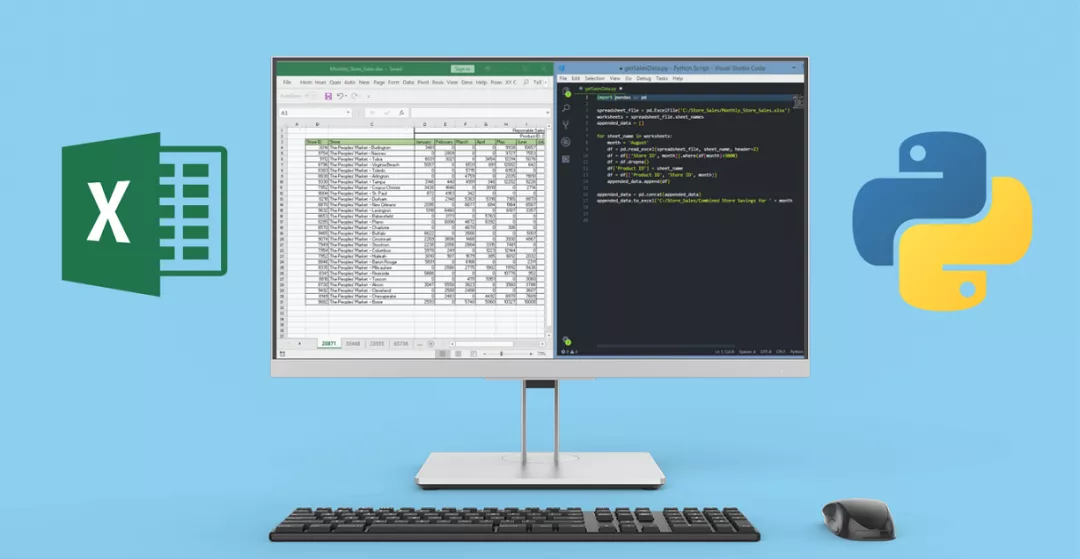##全国校区# 聊聊python 办公自动化之 Excel（上）2020-10-261. 前言

2. 准备

• xlrd / xlwt
• openpyxl
• Pandas
• xlsxwriter
• xlwings
• pywin32

xlrd 和 xlwt 是操作 Excel 文件最多的两个依赖库。其中，xlrd 负责读取 Excel 文件，xlwt 可以写入数据到 Excel 文件，我们安装这两个依赖库。

```# 安装依赖库
pip3 install xlrd
pip3 install xlwt ```

3. xlrd 读取 Excel

```import xlrd

# 打开文件，返回一个工作簿对象
wb = xlrd.open_workbook(file_path)```

```# 统计sheet数量
sheets_num, sheets_names = wb.nsheets, wb.sheet_names()
print('sheet数量一共有:', sheets_num)
print('sheet名称分别为:', sheets_names)```

• 通过 Sheet 名称
• 使用位置索引，从 0 开始
```# 获取某一个sheet
# 通过名称或者索引获取
sheet = wb.sheet_by_index(0)

# sheet = wb.sheet_by_name('第一个Sheet')
print(sheet)```

```# 获取某一个sheet中，包含的行数量、列数量
sheet_name, sheet_row_count,
sheet_column_count = sheet.name, sheet.nrows, sheet.ncols
print('当前sheet名称为:',
sheet_name, ",一共有：",
sheet_row_count, "行；有：",
sheet_column_count, "列")

# 单独获取某一行数据，索引从0开始
# 比如：获取第2行数据
row_datas = sheet.row_values(1)
print('第2行数据为：', row_datas)

# 单独获取某一列数据，索引从0开始
# 比如：获取第二列数据
column_datas = sheet.col_values(1)
print('第2列数据为：', column_datas)```

• 0  --  空（empty）
• 1  --  字符串（string）
• 2  --  数字（number）
• 3  --  date（日期）
• 4  --  boolean（布尔值）
• 5  --  error（错误）
```# 获取某一个单元格的数据
# 比如：获取第2行第1列的单元格的数据
one_cell = sheet.cell(1, 0)
# 单元格的值
cell_value = one_cell.value
print("单元格的值为:", cell_value)
# 单元格数据类型
cell_type = one_cell.
print("单元格数据类型为:", cell_type)```

```# 获取所有单元格的值
print('表格中所有数据如下：')
for r in range(sheet.nrows):
for i in range(sheet.ncols):
print(sheet.cell(r, i).value)```

4. xlwt 写入 Excel

```import xlwt

sheetname = '第一个Sheet'

# 创建一个工作簿对象
wb = xlwt.Workbook()

# 添加Sheet，通过sheet名称

```# 将数据写入到Sheet中
# 3个参数分别是：行索引（从0开始）、列索引（从0开始）、单元格的值
# 第一行第一列，写入一个数据
# 写入标题
for index, title in enumerate(self.titles):
sheet.write(0, index, title)

# 写入值
for index_row, row_values in enumerate(self.values):
for index_column, column_value in enumerate(row_values):
sheet.write(index_row + 1, index_column, column_value)```

```# 保存文件
# 最后保存文件即可
wb.save(filepath)```

5. 进阶用法

1、获取所有可见的 Sheet

```def get_all_visiable_sheets(self, wb):
"""
获取所有可见的sheet
:param wb:
:return:
"""
return list(filter(lambda item: item.visibility == 0, wb.sheets()))

# 1、获取所有可看见的sheet
sheet_visiable = self.get_all_visiable_sheets(wb)
print('所有可见的sheet包含：', sheet_visiable)```

2、获取 Sheet 可见行或列

```def get_all_visiable_rows(self, sheet):
"""
获取某一个sheet中，可见的行
:param sheet:
:return:
"""
result = [index for index in range(sheet.nrows) if sheet.rowinfo_map[index].hidden == 0]
return result

def get_all_visiable_columns(self, sheet):
"""
获取某一个sheet中，可见的列
:param sheet:
:return:
"""
result = [index for index in range(sheet.ncols) if sheet.colinfo_map[index].hidden == 0]
return result```

3、获取单元格的样式

```def get_cell_bg_color(self, wb, sheet, row_index, col_index):
"""
获取某一个单元格的背景颜色
:param wb:
:param sheet:
:param row_index:
:param col_index:
:return:
"""
xfx = sheet.cell_xf_index(row_index, col_index)
xf = wb.xf_list[xfx]

# 字体颜色
font_color = wb.font_list[xf.font_index].colour_index
# 背景颜色
bg_color = xf.background.pattern_colour_index

return font_color, bg_color
```

```# 注意：必须设置formatting_info=True，才能正常获取属性
wb = xlrd.open_workbook(file_path, formatting_info=True)
sheet = wb.sheet_by_index(0```

6. 最后

——热门课程推荐：

OK